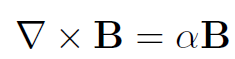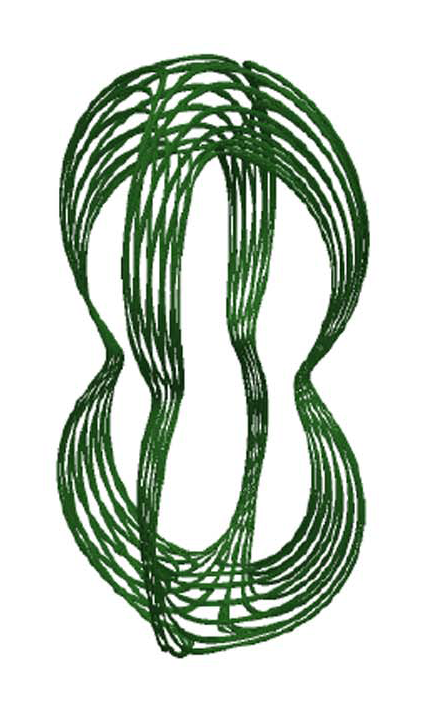## Contact geometry and intergalactic jets

### Chris Prior

BackgroundFigure 1 An example of a force free field, a solution to equation (1), this is a so called magnetic flux rope. Image taken from this nice explainer

We consider a three dimensional vector field B(x) = (B_x(x),B_y(x),B_z(x)) which satisfies the following partial differential equation:with alpha a scalar (often called the Beltrami field or the force free field). The picture you want to keep in mind is that the curl of B represents its rotation as shown above, so this field rotates around itself as shown in Figure 1. There exist a whole family of solutions of this equation which can be written as a Fourier-Bessel series. Remarkably this equation is used to describe fluid vorticies, including tornado's, Reverse pinch magnetic fields used to contain thermonuclear plasmas, and intergalactic jets.Figure 2. Contact geometry: We see local planes, think of them as being normal to the arrows of the direction of the vector B. They cannot be stitched together without the surface overlapping. Figure 3. Surfaces which are contact manifold. The lines pierce the planes. Note how they fold into themselves

It also has another life as a so called Reeb field, the basic structure of a contact geometry. A branch of differential geometry which concerns nowhere-integrable surfaces (sets of planes which fold in on themselves), see Figures 2 and 3 This link has been used to proved the existence of knotted vortex structures in fluids and magnetic field models.This link is actually quite important. For example reverse pinch fields become unstable due to the formation of locally twisted structures (in small regions of the whole field), so called tearing modes. But other more complex force free fields, with interesting internal knotting have been found to be stable, one is shown above.  One can characterise this internal entanglement by the helicity of the field, a measure of its in entanglement (linking), which can also be written as a Fourier-Bessel series.

Project aim

The aim of the project will be to explore how this quantity, the helicity affects the structure and stability of Beltrami fields. It is well known to bound the energy of the system from below so we expect it to control the field's stability and hence use. This will involve exploring the space of Beltrami fields, including knotted configurations described in this paper. One could then perform analytic calculations (stability/energy analyses). Perform numerical calculations of the field's evolution. Or for the more topologically inclined look into aspects of contact topology.

### Prerequisites

None.

From a numerics perspective the Python you have learned will be more than sufficient.

As for courses taking any following modules could be helpful: Fluid dynamics  III,Mathematical Biology III, Dynamical Systems III or Partial Differential Equations III.But not fundamental

### Resources

email: Chris Prior